Linking C MEX S-Function to C++ Code

Today, I am happy to welcome guest blogger Navid Rahbariasr. Navid recently received a request from a user trying to include C++ code in a referenced model in accelerator mode. Unfortunately, this user was forced to use MATLAB R2020b and was not able to take advantage of the new C++ support for model reference simulation target added in MATLAB R2021a. Here is the workaround Navid came up with.
--Guy

Configuring the S-Function Builder to include C++ Code

Let's start with a simple example where our S-Function is calling a method get_a defined in custom C++ source code. The header and the source code for the custom C++ code are as follows: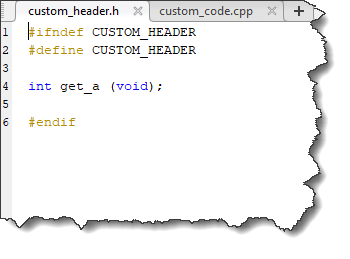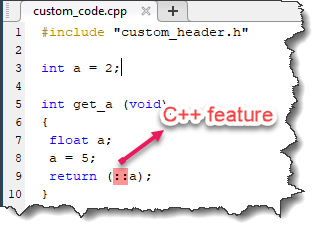Note that "::" is a C++ operator. It is called the scope resolution operator and can be used to access a global variable when there is a local variable with the same name. So, in this example we have a global variable named a, and a local variable with the same name inside the method. By adding :: in front of a we indicate our interest in the global variable a, which in this example has the value of 2. This operator :: is not defined in C, so if you try to compile this code in C, you will get a syntax error.
Now we use the S-Function Builder to create an S-function that calls the get_a function and routes the value to output. In case you did not notice, the S-Function Builder has been entirely revamped in R2020b, allowing you to see the final S-Function code interactively.
We include the custom header in the includes section of the S-Function Builder as shown below: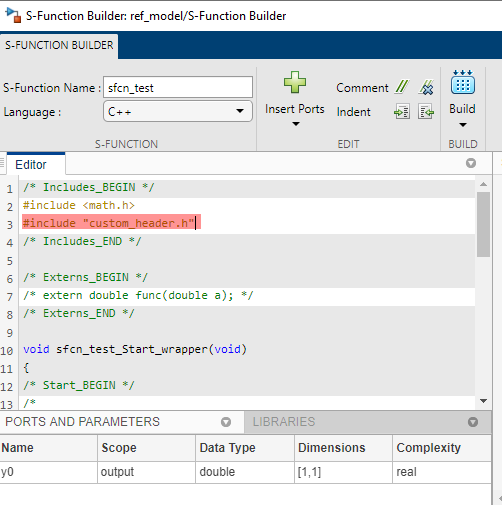We call the method get_a in the S-function outputs wrapper function and assign it to the output of the S-Function block: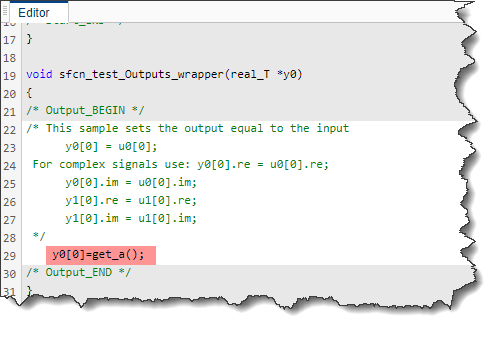We add the C++ source code as a library entry so that S-Function can call the method defined in it. You can find the library entry at the bottom of the S-function Builder interface:And finally, we build the S-Function: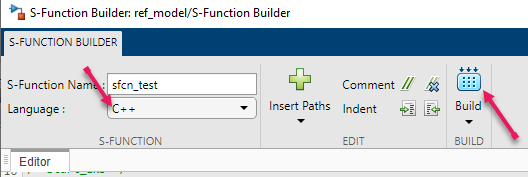Note that we have set the Language of the S-Function to C++. If we set it to C, we will get an error that method get_a is unresolved: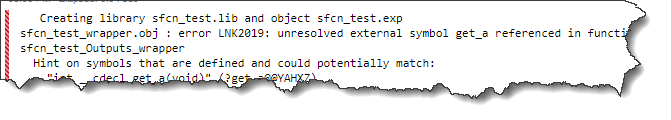By setting the language to C++, the S-function is built. Now if we run the model and check the output, we see the output is 2, the value of the global variable a: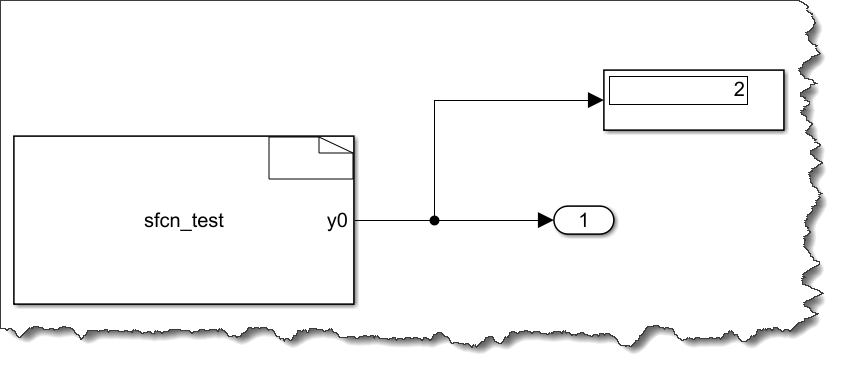Referencing the Model

If we reference the above model in normal mode, we will get the expected value of 2.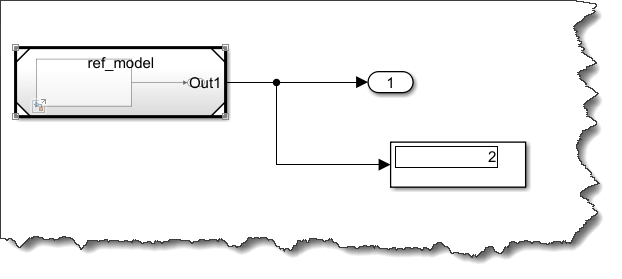However, if we set the model block into accelerator mode and try to run the model, we see an error about an unresolved external symbol that looks quite similar to the one shown above when the S-Function Builder language was set to C instead of C++: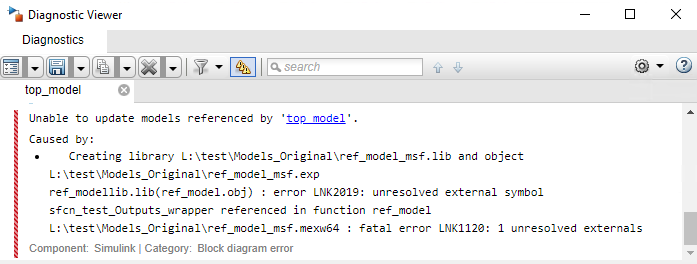The error suggests that Simulink cannot find the sfcn_test_wrapper.c and custom_code.c -- which is accurate because these files have cpp extension rather than c! This is happening because the Simulink engine is first generating a simulation target for the reference model in accelerator mode whose language is C, and thus it is interpreting the symbols as C rather than C++, therefore the accelerator mode build cannot correctly link with the S-Function.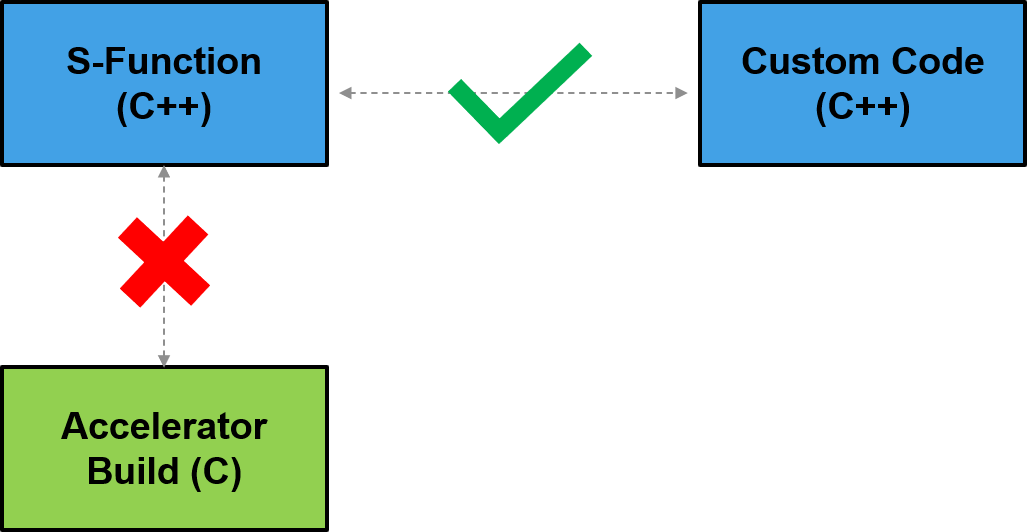This was a limitation of the simulation target in Simulink until R2021a, when C++ support for model reference simulation target has been added.

Workaround

If we want the accelerated referenced model to build successfully, we need to write the S-Functions in C, but our source code is using C++ features, so how we can write the S-Function in C?
The trick is to link the S-function to the compiled version of the source code, so the compiler does not need to recompile it (it is like grinding the C++ food for the C compiler that does not have the right teeth to chew it!) But can C-code call compiled C++ methods? The answer is yes, provided we wrap the C++ declaration methods with extern C {}, so that the compiler uses C naming conventions when compiling the methods.
We first modify our custom header file as below by wrapping the method with extern C: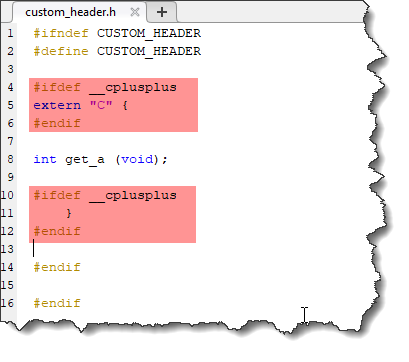Note that #ifdef __cplusplus is a compiler directive. It effectively means "if the language is C++...". When we compile the source code, as the compiler detects it is C++ it wraps the method declaration with extern "C" { } so the compiled object will have C naming schemes.
Now we compile the source code into an object file by using mex with -c flag:
mex -c custom_source.cpp
By running this command, you will get an object file custom_code.obj in the folder which contains the compiled version of the custom_source.cpp: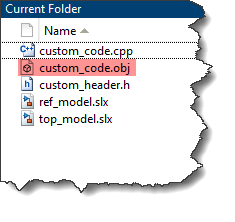Now we go to the S-Function builder and modify two things:
1) Change the language to C
2) Link the S-function to the compiled obj file rather than the uncompiled source file (Surprise! You can link the S-Function to obj files!)As we are linking the S-Function to a compiled C++ code rather than uncompiled C++ code, the S-Function can be built using the C language.
Now if we go to the top model and run the model in accelerated mode, it will build the simulation target successfully and we will get the expected output of 2: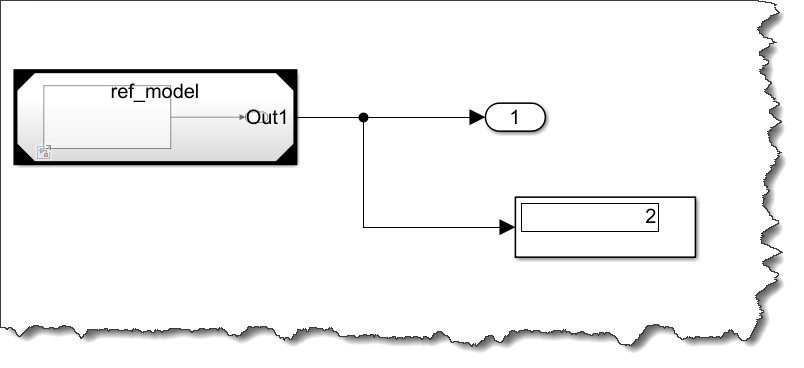Basically, we were able to link the accelerator build which only understands C language to the C MEX S-function and the compiled C++ obj file:C++ support for model reference simulation target in R2021a

The above workaround is useful for older releases. In R2021a, if your model includes C++ S-Functions, you can simply set the Language to C++ in the Simulation Target section of the configuration set, which will allow the accelerator build to understand the C++ language and interpret the C++ S-Function correctly.and everything is built using C++: